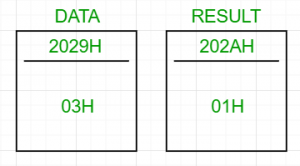# 8085 program to determine if the number is prime or not

Problem – Write an assembly language program for determining if a given number is prime or not using 8085 microprocessor.

If the number is prime, store 01H at the memory location which stores the result, else 00H.

Examples:

```Input : 03H
Output : 01H
The number 3 only has two divisors, 1 and 3.
Hence, it is prime.
Input : 09H
Output : 00H
The number 9 has three divisors, 1, 3 and 9.
Hence, it is composite.
```A prime number is the one which has only two divisors, 1 and the number itself.
A composite number, on the other hand, has 3 or more divisors.

Algorithm:

1. Take n as input
2. Run a loop from i = n to 1. For each iteration, check if i divides n completely or not. If it does, then i is n’s divisor
3. Keep a count of the total number of divisors of n
4. If the count of divisors is 2, then the number is prime, else composite

How to find out if i is a divisor or not?
Keep subtracting i from the dividend till the dividend either becomes 0 or less than 0. Now, check the value of dividend. If it’s 0, then i is a divisor, otherwise it’s not.

Steps:

1. Load the data from the memory location (2029H, arbitrary choice) into the accumulator
2. Initialize register C with 00H. This stores the number of divisors of n
3. Move the value in the accumulator in E. This will act as an iterator for the loop from n to 1.
4. Move the value in the accumulator in B. B permanently stores n because the value in the accumulator will change
5. Move the value in E to D and perform division with the accumulator as the dividend and D as the divisor.
6. Division: Keep subtracting D from A till the value in A either becomes 0 or less than 0. After this, check the value in the accumulator. If it’s equal to 0, then increment the count of divisors by incrementing the value in C by one
7. Restore the value of the accumulator by moving the value in B to A and continue with the loop till E becomes 0
8. Now, move the number of divisors from C to A and check if it’s equal to 2 or not. If it is, then store 01H to 202AH (arbitrary), else store 00H.

202AH contains the result.

2000H LDA 2029H
2001H
2002H
2003H MVI C, 00H
2004H
2005H MOV E, A
2006H MOV B, A
2007H LOOP1 MOV D, E
2008H LOOP2 CMP D
2009H JC DIVIDENDLESSTHAN0
200AH
200BH
200CH SUB D
200DH JNZ LOOP2
200EH
200FH
2010H DIVIDENDLESSTHAN0 CPI 00H
2011H
2013H
2014H
2015H INR C
2017H DCR E
2018H JNZ LOOP1
2019H
201AH
201BH MOV A, C
201CH MVI C, 00H
201DH
201EH CPI 02H
201FH
2020H JNZ COMPOSITE
2021H
2022H
2023H INR C
2024H COMPOSITE MOV A, C
2025H STA 202AH
2026H
2027H
2028H HLT

Store the value of n in 2029H. If 202AH contains 01H, then n is prime, else composite.

Attention reader! Don’t stop learning now. Get hold of all the important CS Theory concepts for SDE interviews with the CS Theory Course at a student-friendly price and become industry ready.

My Personal Notes arrow_drop_upCheck out this Author's contributed articles.

If you like GeeksforGeeks and would like to contribute, you can also write an article using contribute.geeksforgeeks.org or mail your article to contribute@geeksforgeeks.org. See your article appearing on the GeeksforGeeks main page and help other Geeks.

Please Improve this article if you find anything incorrect by clicking on the "Improve Article" button below.